# 0.展示PTA总分（0----2）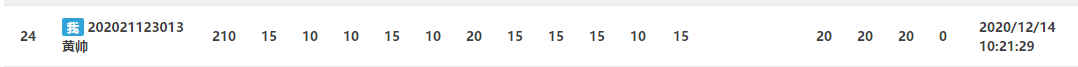# 1.本章学习总结（3分）

## 1.1 指针定义、指针相关运算、指针做函数参数。

• 指针定义
C 语言规定所有变量在使用前必须先定义，指定其类型，并按此分配内存单元。指针变量不同于整型变量和其他类型的变量，它是专门用来存放地址的，所以必须将它定义为“指针类型”。
要注意指针的类型和它所指的类型需要相同。
基类型 *指针变量名;
• 相关运算
将整型变量a的地址赋给整型指针p，使指针p指向变量a。
将a的地址给p1，再将p1的值给p2，所以指针p1与p2都指向a，即p1=p2=a。
赋值：直接对p赋值，如p=0，表示p=NULL
p=4，表示对指针p所指的内容赋值，如 p=a,p=4,表示a=4
• 指针做函数参数
用指针变量作为函数参数, 在函数执行过程中使指针变量所指向的变量值发生变化,函数调用结束后,这些变量值的变化依然保留下来,这样就实现了”通过调用函数使变量的值发生了变化,在主调函数(如main函数)中使用这些改变了值”的目的.

## 1.2 字符指针

• 字符指针：指向字符型数据的指针变量。
• 相关函数（常用）

strcpy strcpy(a,b) 把b字符串内容赋给a字符串 可以使用strncpy能自定义赋值的位数
strcmp strcmp(a,b) 将a串与b串比较 1.a大值为1,b大值为-1,相等值为0. 2.可以使用strncmp自定义比较位数
strcat strcat(a,b) 把b串从头接到a串末尾 可以使用strncat自定义追加位数
strstr strstr(a,b) 在a串中找到b串首次出现的地址 不会包含结束符

## 1.3 指针做函数返回值

• 格式
格式为： 数据类型 *函数名称(形式参数列表) 例如： int *Fun(float x, float y);
返回值为地址。

• 注意事项
函数的返回值类型需要定义成指针变量类型。

## 1.4 动态内存分配

• 为什么要动态内存分配？
动态内存是相对静态内存而言的。所谓动态和静态就是指内存的分配方式。动态内存是指在堆上分配的内存，而静态内存是指在栈上分配的内存。
• 堆区和栈区区别
前面所写的程序大多数都是在栈上分配的，比如局部变量、形参、函数调用等。栈上分配的内存是由系统分配和释放的，空间有限，在复合语句或函数运行结束后就会被系统自动释放。
而堆上分配的内存是由程序员通过编程自己手动分配和释放的，空间很大，存储自由。
• 相关函数
malloc函数：
int *p=NULL;
p=(int *)malloc(2);


calloc函数:

p=(int *)calloc(10,sizeof(int));


## 1.5 指针数组及其应用

• 格式：
类型名 *数组名[数组长度];

• 区别
二维数组：一旦定义，那么每个字符数组的字符串最大长度和首地址都不能改变
字符指针数组:比二维字符数组更加灵活，其指向的每个字符串的首地址可以改变，字符串最大长度也可以改变。

## 1.6 二级指针

• 格式：
类型名 **变量名;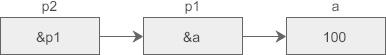## 1.7 行指针、列指针

• 格式：

类型名 (*变量名)[数组长度]


类型名 [数组长度](*变量名)

• 用法
char a;
char (*p);
p=a;
char *q=a;



# 2.PTA实验作业（7分）

## 2.1 藏尾诗（2分）。

### 2.1.1 伪代码

定义整型变量i,j

p=poem
for i=0 to 4
输入每行
end for
for p=poem to poem+4
x=*p的长度
输出 （*p）+x-2
end for


### 2.1.2 代码截图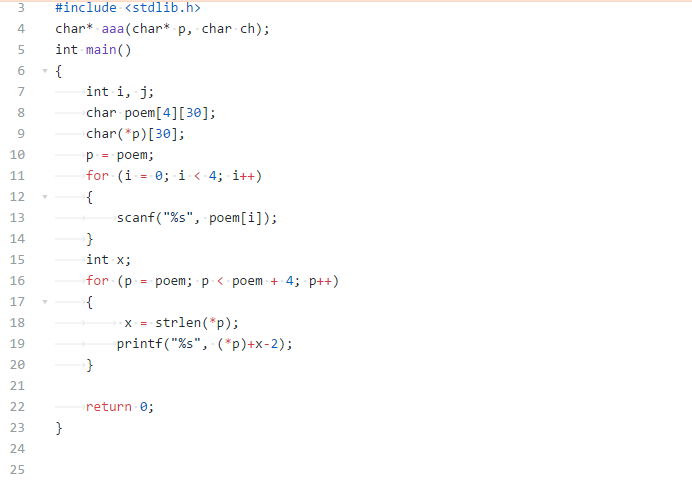### 2.1.3 找一份同学代码比较，说明各自代码特点。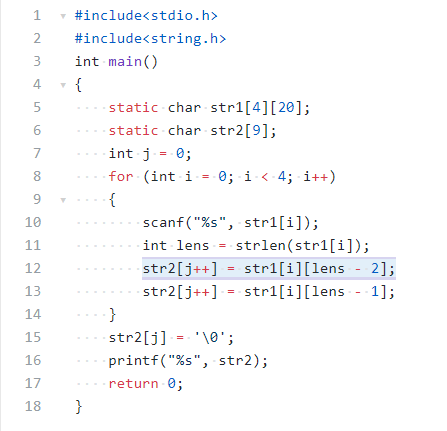## 2.2 选择合并2个有序数组

### 2.2.1 伪代码

int* c
c = (int*)malloc((m + n) * sizeof(int))

i是第一个数组a中的数的顺序
j是第二个数组b中的数的顺序
while k<m+n
if k<m+n 数组a,b中的数都没用完
if a[i] < b[j]
c[k] = a[i]
i++
else
c[k] = b[j]
j++
else
if a用完
c[k] = b[j]
j++
else
c[k] = a[i]
i++
end if
k++
end while



### 2.2.2 代码截图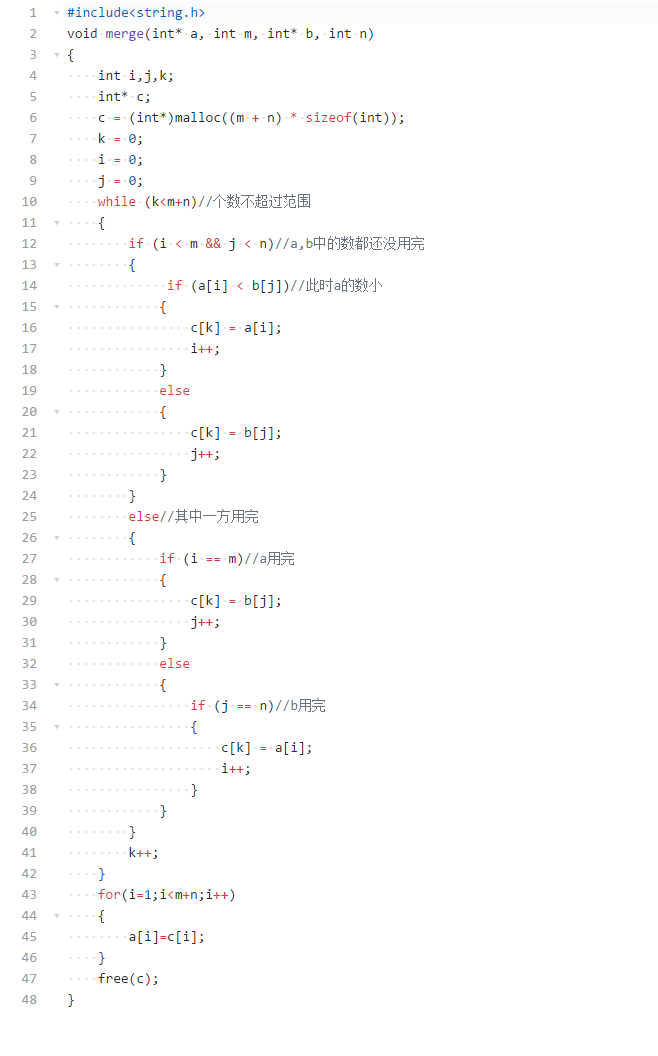### 2.2.3 找一份同学代码比较，说明各自代码特点。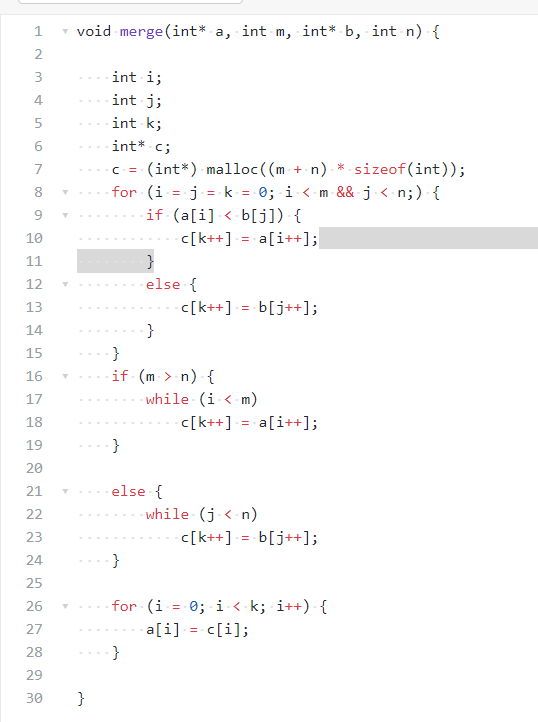## 2.3 反话-加强版

### 2.3.1 伪代码

定义字符型指针变量p,q,a,e

x=strlen(p)
p = (char*)malloc(sizeof(char) * 600000)
for q=p to p+x-2
if *q=空格
for a=q+1 to q+count
输出*a
end  for
if count不为0
flag=1
for e=p to q
if *e不是空格
flag=0
break
end for
if flag为0
输出空格
end if
count=0
end if
else
count++
if q等于p且*p不为空格
for a=q to q+count
输出*a
end for
end if
end for


### 2.3.2 代码截图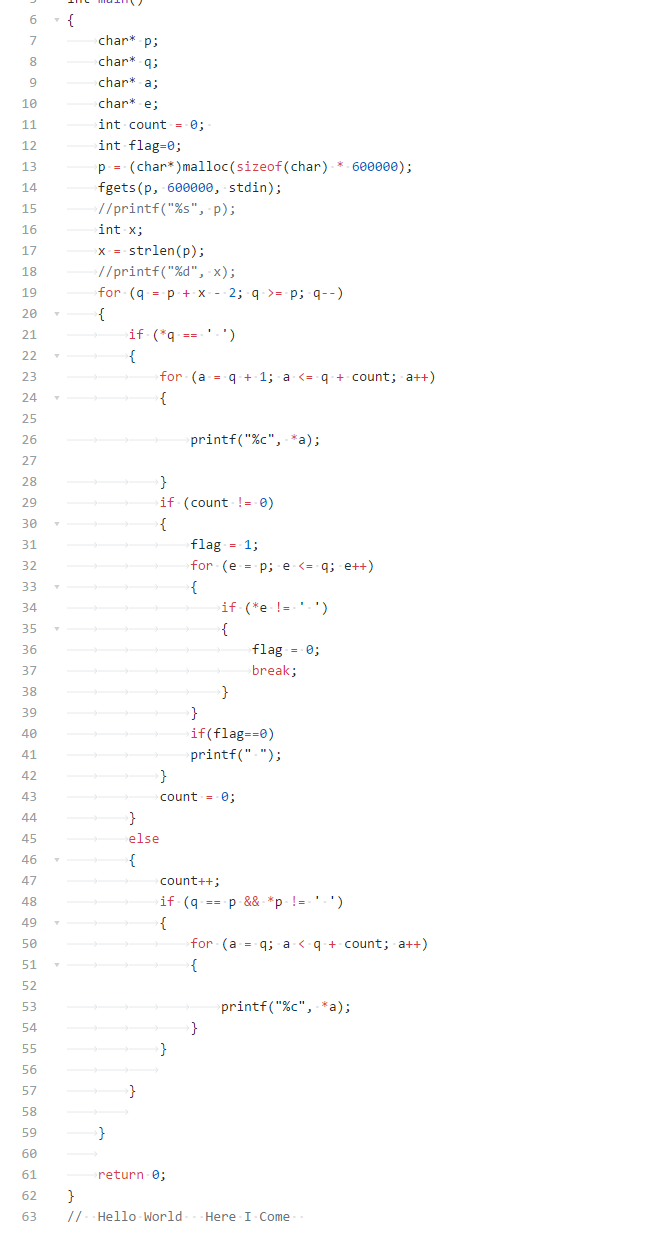### 2.3.3 请说明和超星视频做法区别，各自优缺点。

posted @ 2020-12-27 21:44  黄帅2011  阅读(79)  评论(0编辑  收藏  举报